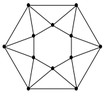# 12 triangles

• MHB
maxkor
There are 12 triangles (picture). We color each side of the triangle in red, green or blue. Among the $3^{24}$ possible colorings, how many have the property that every triangle has one edge of each color?

#### Attachments

•ry.png
2.3 KB · Views: 17

Gold Member
MHB
[TIKZ]\coordinate (A) at (30:2) ;
\coordinate (B) at (90:2) ;
\coordinate (C) at (150:2) ;
\coordinate (D) at (210:2) ;
\coordinate (E) at (270:2) ;
\coordinate (F) at (330:2) ;
\coordinate (U) at (0:5) ;
\coordinate (V) at (60:5) ;
\coordinate (W) at (120:5) ;
\coordinate (X) at (180:5) ;
\coordinate (Y) at (240:5) ;
\coordinate (Z) at (300:5) ;
\foreach \point in {A,B,C,D,E,F,U,V,W,X,Y,Z} \fill [black] (\point) circle (3pt) ;
\draw (A) -- (B) -- (C) -- (D) -- (E) -- (F) -- (A) -- (U) -- (V) -- (W) -- (X) -- (Y) -- (Z) -- (U) --(A) -- (V) -- (B) -- (W) -- (C) -- (X) -- (D) -- (Y) -- (E) -- node[ left ]{$x$}(Z) -- node[ right ]{$z$}(F) -- node[ below ]{$y$}(U) ;
\draw (-90:3.5) node{$6$} ;
\foreach \x in {0,30,...,240} \draw (\x:3.5) node{$2$} ;
\draw (270:2.75) node{$A$} ;
\draw (240:2.75) node{$B$} ;
\draw (0:2.75) node{$C$} ;
\draw (330:2.75) node{$D$} ;
\draw (300:2.75) node{$E$} ;[/TIKZ]
Start with the triangle labelled $A$ at the bottom of the diagram. There are 6 ways to colour its three sides in different colours. Next, look at triangle $B$. One of its sides has already been coloured, and there are 2 ways to colour the remaining sides. Continuing in this way clockwise round the diagram, there are two ways to colour each of the triangles up to and including the one labelled $C$. There are now two cases to consider for the remaining triangles $D$ and $E$. If side $x$ in triangle $A$ and side $y$ in triangle $C$ have different colours then there is only one choice for the colour of side $z$ and therefore only 1 way to colour triangles $D$ and $E$. But if $x$ and $y$ have the same colour then there are two choices for the colour of side $z$, and therefore 2 ways to colour triangles $D$ and $E$.

Here's where the argument becomes probabilistic and unreliable. If the colours of $x$ and $y$ had been chosen independently at random, then the probability of them being the same would be $\frac13$, so the expected number of colourings for triangles $D$ and $E$ would be $\frac13*2 + \frac23*1 = \frac43$. Then the expected value for the number of colourings for the whole diagram would be $$6*2^9 * \frac43 = 2^{12}.$$ But in fact the colourings of $x$ and $y$ are not independent. So the above argument is not rigorous, and the answer may not even be correct (though it must be a good approximation!).

maxkor
[TIKZ]\coordinate (A) at (30:2) ;
\coordinate (B) at (90:2) ;
\coordinate (C) at (150:2) ;
\coordinate (D) at (210:2) ;
\coordinate (E) at (270:2) ;
\coordinate (F) at (330:2) ;
\coordinate (U) at (0:5) ;
\coordinate (V) at (60:5) ;
\coordinate (W) at (120:5) ;
\coordinate (X) at (180:5) ;
\coordinate (Y) at (240:5) ;
\coordinate (Z) at (300:5) ;
\foreach \point in {A,B,C,D,E,F,U,V,W,X,Y,Z} \fill [black] (\point) circle (3pt) ;
\draw (A) -- (B) -- (C) -- (D) -- (E) -- (F) -- (A) -- (U) -- (V) -- (W) -- (X) -- (Y) -- (Z) -- (U) --(A) -- (V) -- (B) -- (W) -- (C) -- (X) -- (D) -- (Y) -- (E) -- node[ left ]{$x$}(Z) -- node[ right ]{$z$}(F) -- node[ below ]{$y$}(U) ;
\draw (-90:3.5) node{$6$} ;
\foreach \x in {0,30,...,240} \draw (\x:3.5) node{$2$} ;
\draw (270:2.75) node{$A$} ;
\draw (240:2.75) node{$B$} ;
\draw (0:2.75) node{$C$} ;
\draw (330:2.75) node{$D$} ;
\draw (300:2.75) node{$E$} ;[/TIKZ]
Start with the triangle labelled $A$ at the bottom of the diagram. There are 6 ways to colour its three sides in different colours. Next, look at triangle $B$. One of its sides has already been coloured, and there are 2 ways to colour the remaining sides. Continuing in this way clockwise round the diagram, there are two ways to colour each of the triangles up to and including the one labelled $C$. There are now two cases to consider for the remaining triangles $D$ and $E$. If side $x$ in triangle $A$ and side $y$ in triangle $C$ have different colours then there is only one choice for the colour of side $z$ and therefore only 1 way to colour triangles $D$ and $E$. But if $x$ and $y$ have the same colour then there are two choices for the colour of side $z$, and therefore 2 ways to colour triangles $D$ and $E$.

Here's where the argument becomes probabilistic and unreliable. If the colours of $x$ and $y$ had been chosen independently at random, then the probability of them being the same would be $\frac13$, so the expected number of colourings for triangles $D$ and $E$ would be $\frac13*2 + \frac23*1 = \frac43$. Then the expected value for the number of colourings for the whole diagram would be $$6*2^9 * \frac43 = 2^{12}.$$ But in fact the colourings of $x$ and $y$ are not independent. So the above argument is not rigorous, and the answer may not even be correct (though it must be a good approximation!).
Almost right :)

Gold Member
MHB
I now think that the answer should be $2^{12} + 2=4098$, but I don't have a proof of that.